# Abstracts

### Hiroki Aoki: Jacobi forms of rational weight and rational index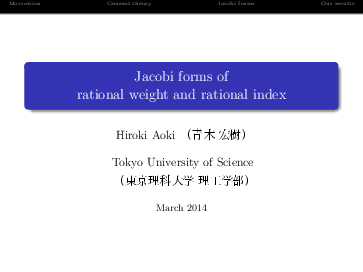On elliptic modular forms, modular forms of rational (not half integral) weight had been studied by Ibukiyama, Bannnai et al. In this talk, we introduce Jacobi forms of rational weight and rational index, from the view point of structure theorem.

### Eiichi Bannai: Spherical designs, modular forms, and toy models for D. H. Lehmer's conjecture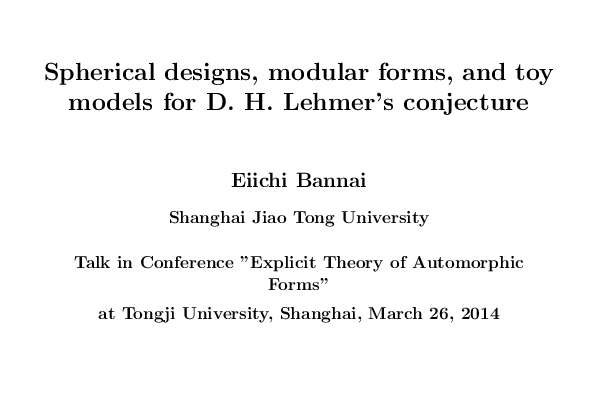A spherical $$t$$-design is a finite subset on the sphere $$S^{n-1}$$ in $$R^n,$$ in which the value of the surface integral of any polynomial $$f$$ of degree at most $$t$$ (divided by the surface area of the sphere) is given exactly by the average value of $$f$$ on the finite set. The relation between spherical $$t$$-designs and modular forms was first explored by Boris Venkov. In particular he noticed that D. H. Lehmer's conjecture that "the value of Ramanujan tau function $$tau(m)$$ is never 0 for any positive integer $$m$$", is equivalent to show that "any shell of 8-dimensional $$E_8$$ root lattice is not a spherical $$8$$-design".
Lehmer's conjecture still remains as a famous open problem. In this talk, we will study so called toy models for Lehmer's conjecture, for several 2-dimentional lattices, instead of the 8-dimentional lattice. Further details will be available in the following papers.
1. Eiichi Bannai and Tsuyoshi Miezaki: Toy models for D. H. Lehmer's conjecture, J. Math. Soc. Japan, 62 (2010), 687-705,
2. Eiichi Bannai and Tsuyoshi Miezaki: Toy models for D. H. Lehmer's conjecture, II, in Quadratic and Higher Degree Forms, (ed. by K. Alladi, et al.), Developments in Mathematics Volume 31, 2013, pp 1--27.
3. E. Bannai, T. Miezaki, and V. A. Yudin, An elementary approach to toy models for D. H. Lehmer's conjecture (Russian), Izvestiya RAN: Ser. Mat.75:6 (2011) 3--16, (translation: Izv. Math. 75 (2011), 1093--1106.)

### Siegfried Boecherer: Growth of Fourier coefficients of noncusp forms

If the Fourier expansion of a Siegel modular form on an arbitrary congruence subgroup satisfy a "Hecke bound" in some cusp, then the modular form is cuspidal. We show this by analyzing the growth of Hecke eigenvalues by purely local algebraic methods (joint work with S.Das)

### Jan Bruinier: Modularity of special cycles and formal Fourier Jacobi series

A famous theorem of Gross, Kohnen, and Zagier states that Heegner divisors on modular curves are the coefficients of weight 3/2 modular forms with values in the first Chow group. A far reaching conjecture of Kudla predicts more generally that special cycles of codimension r on Shimura varieties associated to orthogonal groups of signature of signature (n,2) are the coefficients of Siegel modular forms of genus r with values in the r-th Chow group. We report on older results on the subject, and on more recent work by W. Zhang, M. Raum and myself. These results involve modularity theorems for vector valued formal Fourier-Jacobi series.

### Yingchun Cai: Some results in additive prime number theory

In this report we outline some results in additive prime number theory obtained by incorporating the Hardy-Littlewood method into the sieve theory.

### Neil Dummigan: A Harder conjecture for spinor L-values

Harder's conjecture is a congruence between Hecke eigenvalues of a vector-valued Siegel cusp form of genus two and a Langlands Eisenstein series attached to a cusp form of genus one, modulo a divisor of a critical value of the L-function of the latter. This is an instance of a very general conjecture on automorphic representations of reductive groups. We will look at another instance, in which the genus one form and its L-function are replaced by a genus two form and its spinor L-function. We will also consider a half-integral weight version, and speculate on how it might be proved, and spinor L-values calculated.

### Stephan Ehlen: Simple lattices of signature (2,n)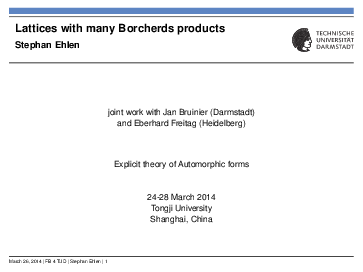We give a complete classification of all isomorphism classes of lattices of signature (2,n) such that the space of vector valued cusp forms of weight (2+n)/2 vanishes. This space is the space of obstructions for the Borcherds lift. If it vanishes, every linear combination of Heegner divisors is a principal divisor.

### Jens Funke: Cohomolgical aspects of weakly holomorphic modular forms and periods

In this talk we give a simple cohomological identity between two weakly holomorphic forms obtained by applying certain differential operators to a given harmonic Maass form and derive several consequences.

### Alexandru Edgar Ghitza: Distinguishing Hecke eigenforms and irreducibility of Hecke polynomials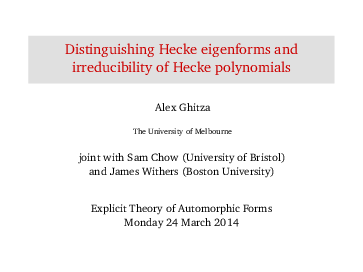A popular way of specifying a modular form is by giving its Fourier expansion. As this is an infinite power series, for practical purposes we are interested in questions such as: given two eigenforms, how many Fourier coefficients do we have to compare in order to distinguish the forms? I will describe some theoretical and computational results, and give a simple relation between this question and the properties of the Hecke polynomials of spaces of modular forms. Most of the work is joint with Sam Chow (Bristol) and James Withers (Boston University).

### Shuichi Hayashida: Lifts from two elliptic modular forms to Siegel modular forms of half-integral weight of even degrees.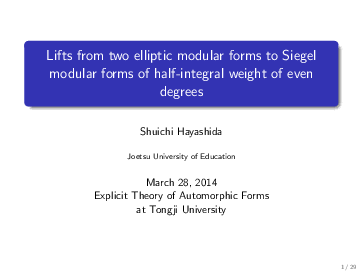In this talk I will explain lifts from two elliptic modular forms to Siegel modular forms of half-integral weight of even degrees under the assumption of the non-vanishing of such lifts.

### Hidenori Katsurada: Refined version of Ikeda's conjecture on the period of the Hermitian Ikeda lift and its applicationIn [Copmpositio Math. 144(2008), 1107-1154], Ikeda constructed a lifting from an elliptic modular form to a Hermitian modular form, which we call the Hermitian Ikeda lift. Moreover he proposed a conjecture on the period of the Hermitian Ikeda lift. About four years ago, I prove his conjecture with a minor modification. In this report I discuss its refined version, from which I can prove its adelic version. If time permits, I talk about an application of our result to congrueces of the adelic Hermitain Ikeda lifts.

### Hidetaka Kitayama: On Siegel modular forms with respect to non-split symplectic groups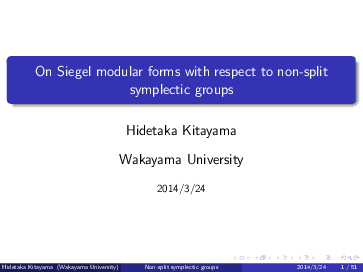In this talk, we first consider explicit studies for Siegel modular forms with respect to non-split symplectic groups of degree two, especially explicit dimension formulas and ring structures. After that, as applications of these results, we also discuss some conjectures on correspondences and liftings, a large part of which is in a joint paper with Ibukiyama.

### Winfried Kohnen: Explicit bounds for the first sign change of the Fourier coefficients of a Siegel cusp form

We will give an explicit bound for the first sign change of the Fourier coefficients (when real) of a Siegel cusp form of arbitrary degree n. This is very recent joint work with YoungJu Choie and Sanoli Gun.

### Jianya Liu: Explicit error term in the prime number theorem for Rankin-Selberg L-functions

For any two automorphic cuspidal representations of GL(m), one can attach a Rankin-Selberg L-function. In the special case when one of these representations is self-contragredient, a prime number theorem, with error term of de la Vallee Poussin's classical type, can be established. If, however, none of them is self-contragredient, then the error term known is very weak. In this talk I will explain how an explicit error term can be obtained, which is particularly stronger in the general case when none of these representations is self-contragredient.

### Guangshi Lu: Shifted convolution of cusp-forms with $$\theta$$-series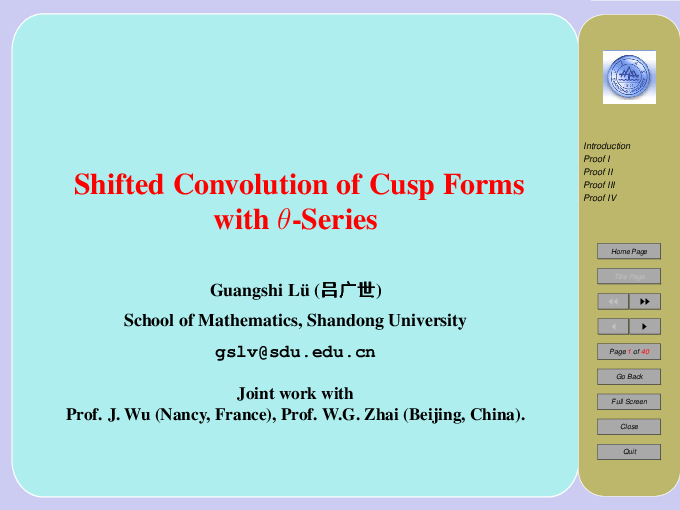In this talk, we introduce a simple approach to study a shifted convolution sum involving the Fourier coefficients of cusp forms with those of theta series.

### Murugesan Manickam: On newforms of half-integral weight

In this talk I present my recent work on theory of newforms of half-integral weight for both plus space and full space for the group $$\Gamma_0(2^a N)$$. Where $$a=3,4$$,$$N$$ is an odd square free integer.

### Alexei Pantchichkine: Computing L-values and Peterson products via algebraic and p-adic modular forms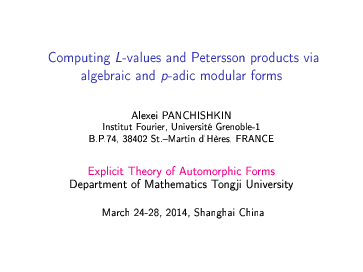The use of the Gauss-Manin connection and the Maass-Shimura differential operator for computing with nearly-holomorphic Siegel modular forms and proving congruences between them are discussed. Constructing p-adic measures via congruences between algebraic modular forms using the canonical projection method is explained. Doubling method and Rankin-Selberg method and their algebraic and p-adic versions are used.

### Hourong Qin: On the representation numbers of ternary quadratic forms and modular forms of weight $$3/2$$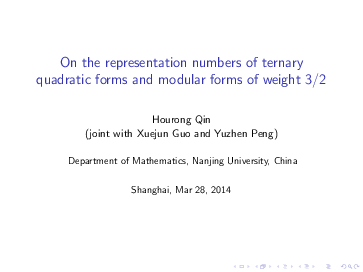We use the explicit basis of the Eisenstein spaces of weight 3/2 to give an alternative proof of Berkovich and Jagy's genus identity and verify a series of conjectures raised by Cooper and Lam on the number of solutions of $$n^2=x^2+by^2+cz^2$$ and two conjectures raised by Sun on the number of solutions of $$p=x^2+y^2+3z^2$$, $$3p=x^2+y^2+3z^2$$. This is joint work with Xuejun Guo and Yuzhen Peng.

### B. Ramakrishnan: Jacobi forms and differential operators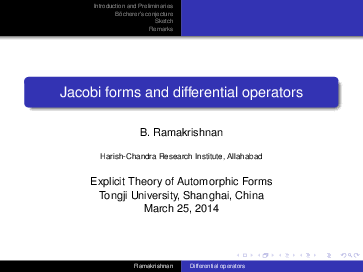In this talk, we report on our joint work with Soumya Das about the question of Bocherer about the feasibility of removing one di erential operator from the standard collection of m+1 of them used to embed the space of Jacobi forms of weight 2 and index m into several pieces of elliptic modular forms.

### Hiroshi Sakata: Some remarks on the trace formula for Jacobi forms of prime power level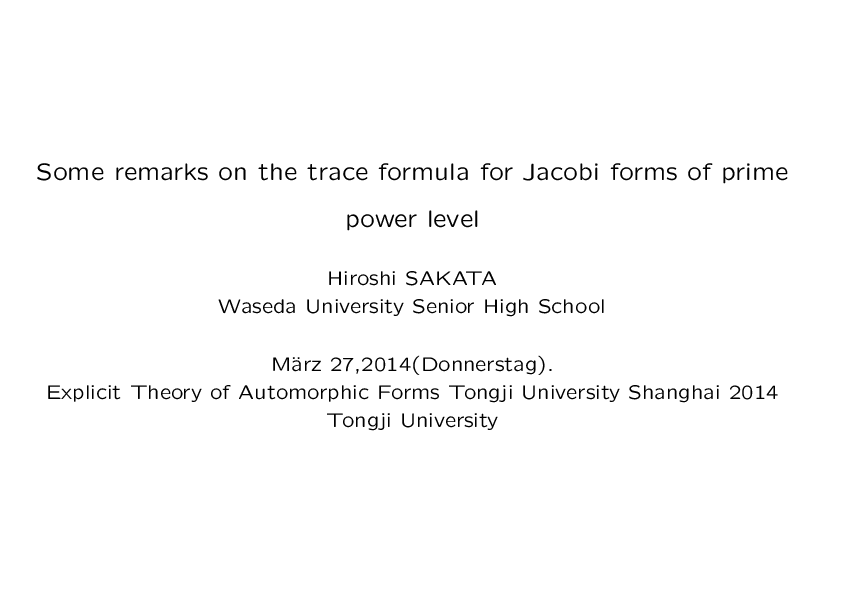We describe the trace formulas for Jacobi forms of prime power level, and give some trace relations between them and the trace formulas for elliptic modular forms. Moreover we consider about the level-index changing operator (the `swapping' operator) on Jacobi forms in the case of prime odd power level.

### Rainer Schulze-Pillot: Modular forms for some generalized Fricke groups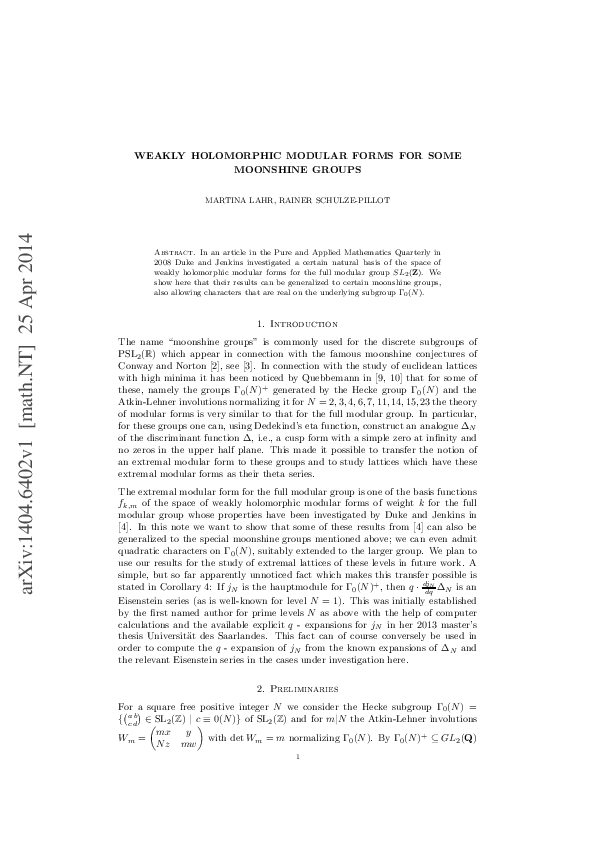We generalize results of Duke and Jenkins for weakly holomorphic modular forms for the full modular group to the setting of certain generalized Fricke groups. This is joint work with Martina Lahr (Master thesis).

### Fredrik Stromberg: Dimension formulas for vector-valued Hilbert modular forms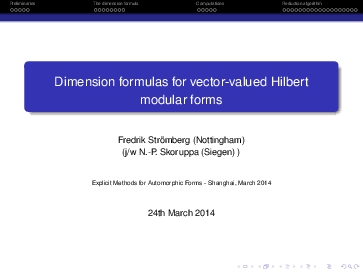In ongoing work joint with N.P. Skoruppa we are working on explicit dimension formulas for vector-valued Hilbert modular forms. In the current talk I will give a brief overview including the main results. I will also discuss the main computational challenges and present some of our solutions to these challenges.

### Ye Tian: Cube Sums

In this talk, we prove that for any given positive integer k, there are infinitely many cube free integers which are sum of two rational cubes and have exactly k prime factors.

### Lynne Walling: Hecke operators on Siegel Eisenstein series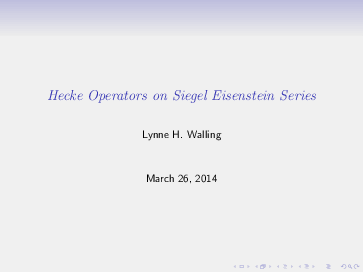Classically, we evaluate the action of Hecke operators on elliptic modular forms using a Fourier series expansion for the form. However, in the case of Siegel Eisenstein series of arbitrary level, we do not have formulas for the Fourier coefficients of all the Eisenstein series. Using an explicit set of matrices giving the action of the Hecke operators on Siegel modular forms, we evaluate the action of the Hecke operators on a basis of Siegel Eisenstein series. We consider integral and half-integral weights.

### Linsheng Yin: Derivative of Siegel modular forms and Jacobi forms by connections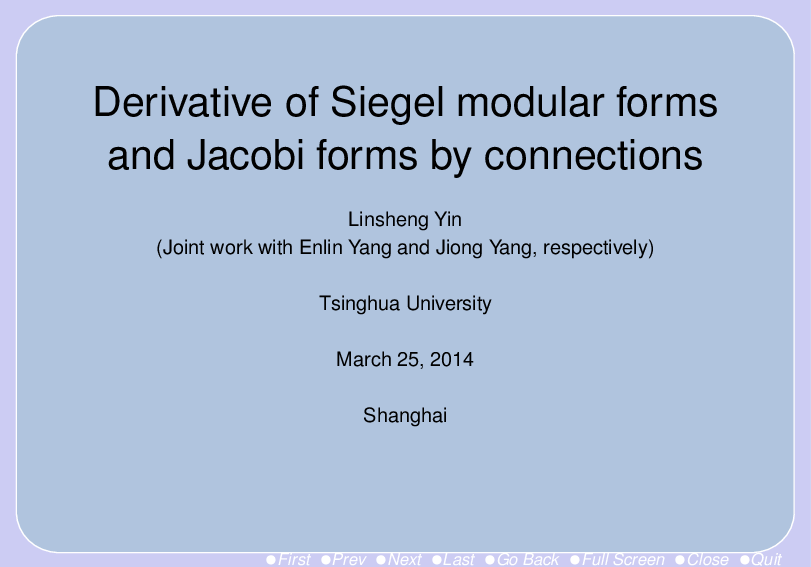We will construct a series of differential operators of Siegel modular forms and Jacobi form by computing the Levi-Civita connections on the Siegel upper half plane and on the Siegel-Jacobi space, respectively.

### David S. Yuen: Paramodular Forms and Borcherds Products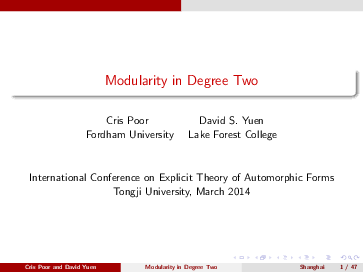We use Borcherds Products to construct paramodular forms. In particular, we construct paramodular new forms that give evidence for the Paramodular Conjecture.